# GCSE Maths Paper 02 (Foundation) Practice Paper in the Style of OCR - Non Calculator

In this assessment, students will be able to complete a timed GCSE Mathematics Paper 02 (Non-Calculator) in the style of OCR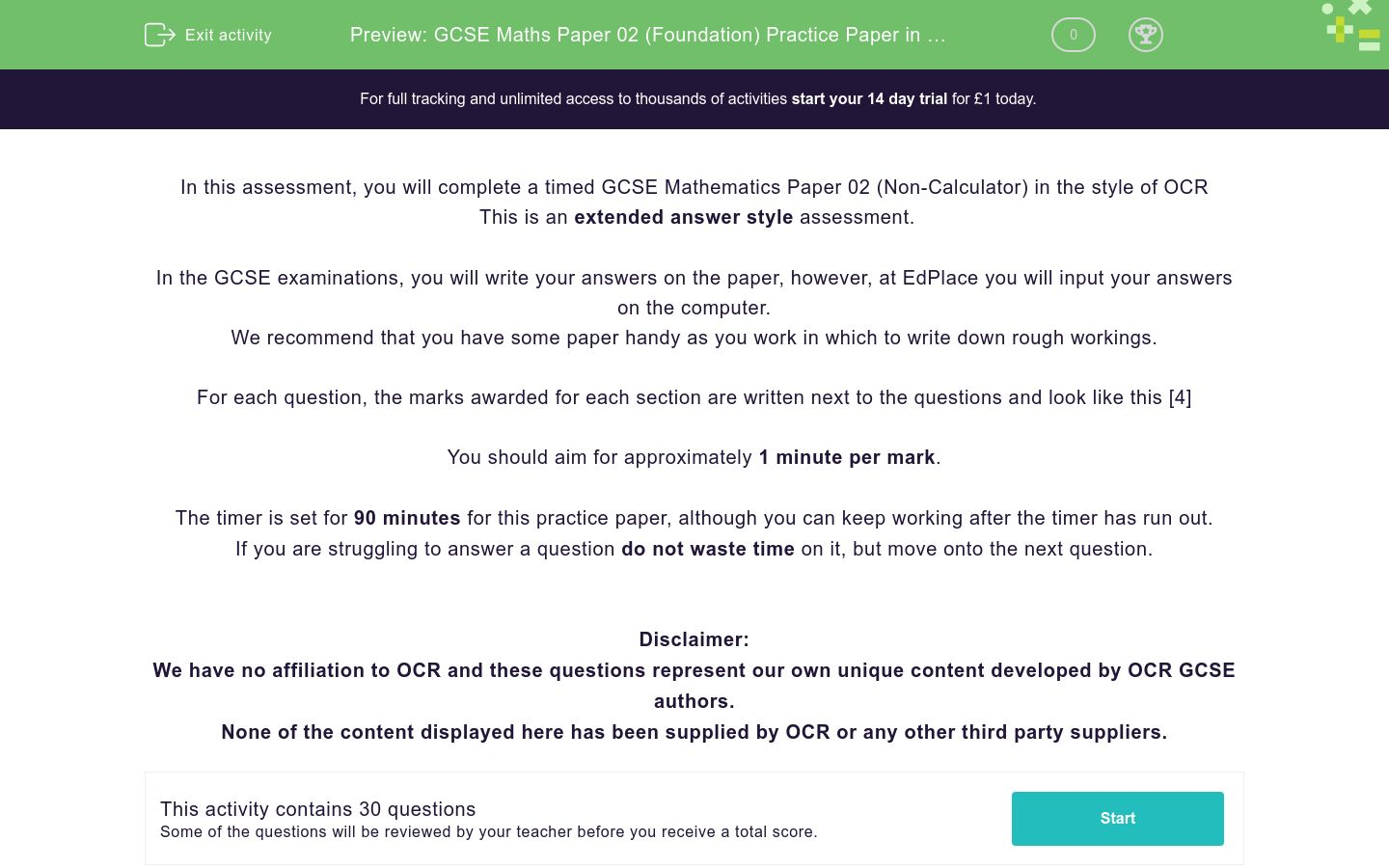Key stage:  KS 4

GCSE Subjects:   Maths

GCSE Boards:   OCR

Curriculum topic:   Foundation Practice Papers

Curriculum subtopic:   Non-Calculator Practice Papers

Difficulty level:### QUESTION 1 of 10

In this assessment, you will complete a timed GCSE Mathematics Paper 02 (Non-Calculator) in the style of OCR

This is an extended answer style assessment.

In the GCSE examinations, you will write your answers on the paper, however, at EdPlace you will input your answers on the computer.

We recommend that you have some paper handy as you work in which to write down rough workings.

For each question, the marks awarded for each section are written next to the questions and look like this 

You should aim for approximately 1 minute per mark.

The timer is set for 90 minutes for this practice paper, although you can keep working after the timer has run out.

If you are struggling to answer a question do not waste time on it, but move onto the next question.

Disclaimer:

We have no affiliation to OCR and these questions represent our own unique content developed by OCR GCSE authors.

None of the content displayed here has been supplied by OCR or any other third party suppliers.

Write 0.62 as a percentage.



Write down a prime number between 10 and 20



Work out 7 x (2+1)



What number is exactly half-way between 21 and 33?



Write 150 seconds in minutes



Point A is shown on this grid.(a) Write down the coordinates of point A.              

(b) (i) Plot point B at (4,1)             

(ii) Does point B lie on the line y = 3x – 2? Give a reason for your answer.          

(c) Draw the line y = 5 on the grid.            

Jamie has 3 dogs.

Each one eats ¾ of a tin of dog food each day.

She buys 10 tins of dog food.

Does she have enough to feed her dogs for 4 days?



Calculate,

(a)(b)Stephen left home at 6 am and cycled to work. He got to work at 06:30 and was cycling at an average speed of 12 mph.

(a) Find the distance Stephen cycled.            

Stephen worked for 9 hours and then cycled home. He took 50 minutes to cycle home.

(b) What time did Stephen get home?              

Jonny drives to a friend’s wedding in France with 3 friends.

The costs are…

£600 for 4 return ferry tickets

£650 to hire a villa to stay in

£200 for petrol for his car

The four friends split the cost equally. What is Jonny’s share?



Here are some fractions.One of these fractions is not equal to 2/3.

(a) Which fraction?                 

(b) Work out 1/4 + 2/3             

There are 30 pupils in a school class.

18 of the pupils are male

8 of the female students are right-handed

12 of the male students are left-handed.

(a) Use this information to complete the two-way table.



 Male Female Total Left-handed Right-handed Total

One of the students is chosen at random.

(b) What is the probability that this is a left-handed girl?



The total surface area of a cube is 150 cm2.

Find the volume of the cube.



James, John and Jim all earn the same each month.

James spends 65% of his salary and saves the rest.

John spends 34/50 of his salary and saves the rest.

Jim spends and saves his salary in the ratio 3:2

Work out who saves the least of their salary each month.



Two shapes are shown below, a triangle and a rectangle.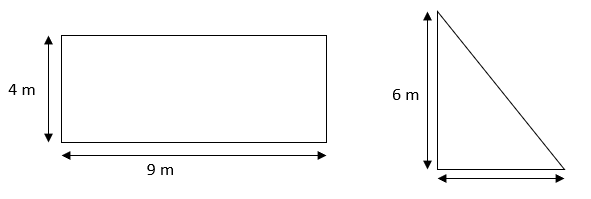The rectangle has an area three times bigger than the area of the triangle.

Show that the length of the base of the triangle is 4 cm.



A car is travelling with a velocity of 10 ms-1.

It then accelerates at 3 ms-2​ for 2 seconds.

Use the formula

s = ut + ½ at2

to find the distance travelled while the car is accelerating.



5 apples cost £1.55

3 apples and 4 oranges cost £2.41

Work out the cost of 2 apples and 3 oranges.



The Food Store and Caffeine Central both sell the same type of coffee.

Each shop has a special offer;

The Food Store.

500 g costs £3 = 25% off the price

Caffeine Central

500 g cost £3 = Extra 25% for the same price

Which jar of coffee is the better value for money?

Show how you decide?



a) Work outb) Work outIn a bag, the number of squares and circles are in the ratio 3:7.

The number of circles and triangles are given in the ratio 14:9.

If there are 36 squares in the bag, how many triangles are in the bag?



Work out 36.2 x 4.9                                            

a) A Formula One car travels at 182.9 miles per hour. Find an estimate of the number of miles travelled in 1 minute.        

Six apples and 5 bananas cost £3.80

Four apples and 6 bananas cost £3.60

Find the cost of 3 apples and 4 bananas.

[3[

Here are two shapes, a rectangle and a triangle that have the same perimeter.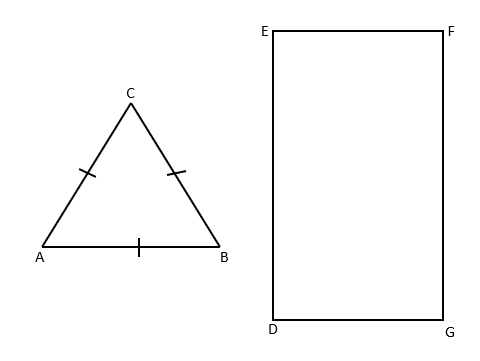The length of the side FG is 10 cm

The area of the rectangle DEFG is 50 cm2

Find the length of the side AB



a) Write down the exact value of sin 60        

b) Here is a right-angled triangle.Cos 30 = 0.5

Find the value of x.          

The size of each interior angle of a regular polygon is 3 times the size of each exterior angle.

What is the name of this regular polygon?



(a) Simplify.

(i) 3x + 2y – x + 4y            

(ii) p7 ÷ p3                              

(iii) 4a2b x 3ab3                  

(b) Work out the value of

(i) 3x + 6 when x = 2           

(ii) 5x – 4 when x = -3           

(a) Multiply out.

3a(b – 2)                    

(b) Multiply out and simplify.

(2x + 3)(x – 2)            

(c) Solve.

4x – 1 ≥ 23                  

The table shows the relative frequencies of the results for a rugby team after a number of games.

 Result Win Loss Draw Relative Frequency 0.12 0.64

(a) Complete the table           

(b) The team drew 13 more matches than they won.

How many games did they play altogether?



The fourth and fifth term of a Fibonacci sequence are 2a + 2b and 4a + 3b

……….     ……….     ……….     2a + 2b     4a + 3b

Write down algebraic expressions for the first, second and third terms.



• Question 1

Write 0.62 as a percentage.



62
62%
62 %
EDDIE SAYS
Nice and easy one to start with. Remember the rule that to change a decimal into a percentage, you just multiply it by 100.
• Question 2

Write down a prime number between 10 and 20



11
13
17
19
EDDIE SAYS
A prime number is just a number that can only be divided by itself and 1. The only numbers that fit this description between 10 and 20 are 11, 13, 17 and 19. Any of these will get you the mark.
• Question 3

Work out 7 x (2+1)



21
EDDIE SAYS
A nice little BODMAS question. Did you spot the bracket? Remember this means you have to do this first.
• Question 4

What number is exactly half-way between 21 and 33?



27
EDDIE SAYS
There's a really easy way to do this. Add them together and then half it. Give it a try.
• Question 5

Write 150 seconds in minutes



2.5
EDDIE SAYS
The biggest mistake people make here is to put 2.30. Remember that 30 seconds is 0.5 minutes.
• Question 6

Point A is shown on this grid.(a) Write down the coordinates of point A.              

(b) (i) Plot point B at (4,1)             

(ii) Does point B lie on the line y = 3x – 2? Give a reason for your answer.          

(c) Draw the line y = 5 on the grid.            

EDDIE SAYS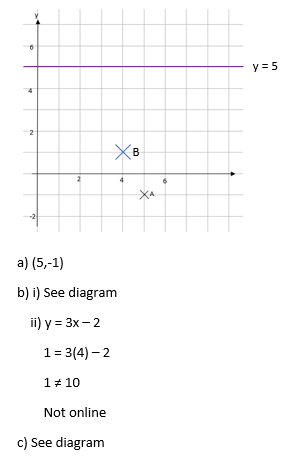• Question 7

Jamie has 3 dogs.

Each one eats ¾ of a tin of dog food each day.

She buys 10 tins of dog food.

Does she have enough to feed her dogs for 4 days?



EDDIE SAYS• Question 8

Calculate,

(a)(b)EDDIE SAYS• Question 9

Stephen left home at 6 am and cycled to work. He got to work at 06:30 and was cycling at an average speed of 12 mph.

(a) Find the distance Stephen cycled.            

Stephen worked for 9 hours and then cycled home. He took 50 minutes to cycle home.

(b) What time did Stephen get home?              

EDDIE SAYS• Question 10

Jonny drives to a friend’s wedding in France with 3 friends.

The costs are…

£600 for 4 return ferry tickets

£650 to hire a villa to stay in

£200 for petrol for his car

The four friends split the cost equally. What is Jonny’s share?



EDDIE SAYS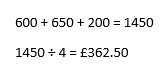• Question 11

Here are some fractions.One of these fractions is not equal to 2/3.

(a) Which fraction?                 

(b) Work out 1/4 + 2/3             

EDDIE SAYS• Question 12

There are 30 pupils in a school class.

18 of the pupils are male

8 of the female students are right-handed

12 of the male students are left-handed.

(a) Use this information to complete the two-way table.



 Male Female Total Left-handed Right-handed Total

One of the students is chosen at random.

(b) What is the probability that this is a left-handed girl?



EDDIE SAYS• Question 13

The total surface area of a cube is 150 cm2.

Find the volume of the cube.



EDDIE SAYS• Question 14

James, John and Jim all earn the same each month.

James spends 65% of his salary and saves the rest.

John spends 34/50 of his salary and saves the rest.

Jim spends and saves his salary in the ratio 3:2

Work out who saves the least of their salary each month.



EDDIE SAYS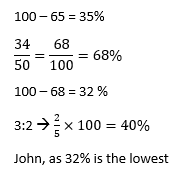• Question 15

Two shapes are shown below, a triangle and a rectangle.The rectangle has an area three times bigger than the area of the triangle.

Show that the length of the base of the triangle is 4 cm.



EDDIE SAYS• Question 16

A car is travelling with a velocity of 10 ms-1.

It then accelerates at 3 ms-2​ for 2 seconds.

Use the formula

s = ut + ½ at2

to find the distance travelled while the car is accelerating.



EDDIE SAYS• Question 17

5 apples cost £1.55

3 apples and 4 oranges cost £2.41

Work out the cost of 2 apples and 3 oranges.



EDDIE SAYS• Question 18

The Food Store and Caffeine Central both sell the same type of coffee.

Each shop has a special offer;

The Food Store.

500 g costs £3 = 25% off the price

Caffeine Central

500 g cost £3 = Extra 25% for the same price

Which jar of coffee is the better value for money?

Show how you decide?



EDDIE SAYS• Question 19

a) Work outb) Work outEDDIE SAYS• Question 20

In a bag, the number of squares and circles are in the ratio 3:7.

The number of circles and triangles are given in the ratio 14:9.

If there are 36 squares in the bag, how many triangles are in the bag?



EDDIE SAYS• Question 21

Work out 36.2 x 4.9                                            

EDDIE SAYS• Question 22

a) A Formula One car travels at 182.9 miles per hour. Find an estimate of the number of miles travelled in 1 minute.        

EDDIE SAYS• Question 23

Six apples and 5 bananas cost £3.80

Four apples and 6 bananas cost £3.60

Find the cost of 3 apples and 4 bananas.

[3[

EDDIE SAYS• Question 24

Here are two shapes, a rectangle and a triangle that have the same perimeter.The length of the side FG is 10 cm

The area of the rectangle DEFG is 50 cm2

Find the length of the side AB



EDDIE SAYS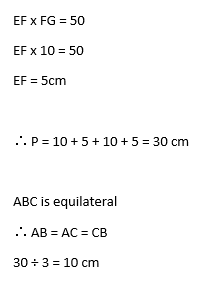• Question 25

a) Write down the exact value of sin 60        

b) Here is a right-angled triangle.Cos 30 = 0.5

Find the value of x.          

EDDIE SAYS• Question 26

The size of each interior angle of a regular polygon is 3 times the size of each exterior angle.

What is the name of this regular polygon?



EDDIE SAYS• Question 27

(a) Simplify.

(i) 3x + 2y – x + 4y            

(ii) p7 ÷ p3                              

(iii) 4a2b x 3ab3                  

(b) Work out the value of

(i) 3x + 6 when x = 2           

(ii) 5x – 4 when x = -3           

EDDIE SAYS• Question 28

(a) Multiply out.

3a(b – 2)                    

(b) Multiply out and simplify.

(2x + 3)(x – 2)            

(c) Solve.

4x – 1 ≥ 23                  

EDDIE SAYS• Question 29

The table shows the relative frequencies of the results for a rugby team after a number of games.

 Result Win Loss Draw Relative Frequency 0.12 0.64

(a) Complete the table           

(b) The team drew 13 more matches than they won.

How many games did they play altogether?



EDDIE SAYS• Question 30

The fourth and fifth term of a Fibonacci sequence are 2a + 2b and 4a + 3b

……….     ……….     ……….     2a + 2b     4a + 3b

Write down algebraic expressions for the first, second and third terms.



EDDIE SAYS